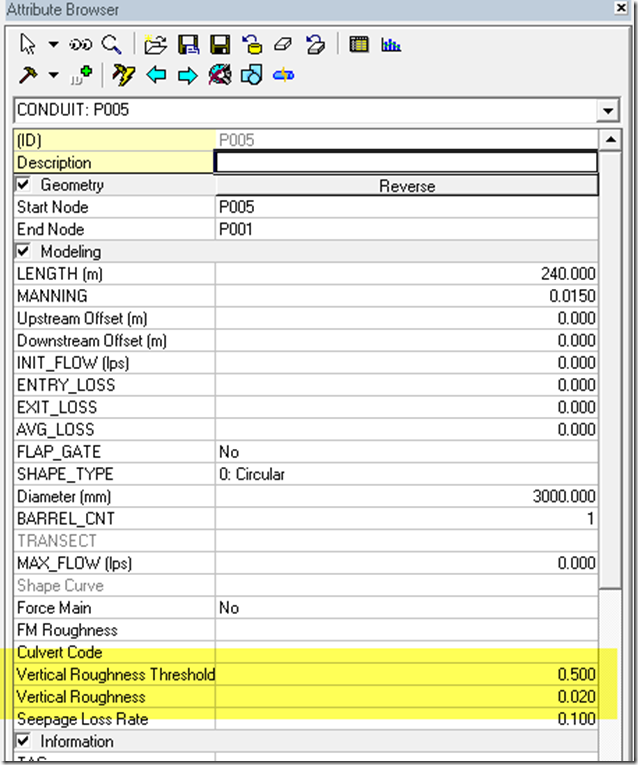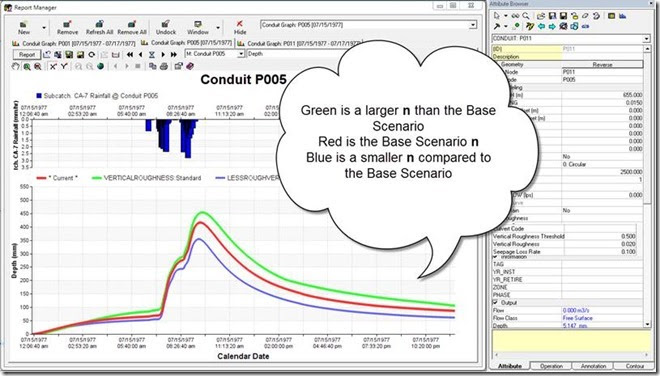## Tuesday, February 10, 2015

### Vertical Roughness Differentiation in InfoSWMM for a Link

The Vertical Roughness Threshold allows the user of InfoSWMM or H2OMap SWMM to have a simple differentiation of roughness in a link. The Threshold depth which is entered as a fraction of the total link depth allows the simulation of lower or higher roughness at the bottom of the link compared to the top of the link. The lower or higher roughness is entered as the Vertical Roughness value in the InfoSWMM Attribute Table of DB Table for the link hydraulic data.

1. The Vertical Roughness Threshold is a fraction and the Threshold depth calculated from this fraction will be the Vertical Roughness Threshold Fraction * Maximum depth. If the Maximum depth is 3 meters and the Vertical Roughness Threshold is 0.5 then the dividing depth is 1.5 meter (Figure 1 and Figure 2).
2. If the depth in the link is higher than the Threshold depth it will use the link manning roughness n value. If the depth is in the link during the simulation is less than the Threshold depth it will use the Vertical Roughness n value (Figure 2).
3. For example in Figure 3, I am showing the Base Scenario (Blue line), a Scenario with a larger Vertical Roughness n value (Green Line) and a Scenario with a smaller Vertical Roughness n value (Red Line). The larger the Vertical Roughness n value the larger the simulated depth in the link for the same flow input.Figure 1.  The Vertical Roughness Threshold and Vertical Roughness Manning’s n value in the Attribute Browser of InfoSWMM and H2OMap SWMM.Figure 3.  A Three Scenario Comparison of the Effect of Vertical Roughness.  The Vertical Roughness can be either rougher or less rougher than the default link Manning’s n.